Question

# Given a list of integers, return a list where each integer is added to 1 and...

Given a list of integers, return a list where each integer is added to 1 and the result is multiplied by 10. You must use a list comprehension

math1([1, 2, 3]) → [20, 30, 40]

math1([6, 8, 6, 8, 1]) → [70, 90, 70, 90, 20]

math1() → 

Complete in Python code the starter code is listed bellow

def math1(lst):

return lst

print(math1([1, 2, 3])) # [20, 30, 40]
print(math1([6, 8, 6, 8, 1])) # [70, 90, 70, 90, 20]
print(math1()) # 

def math1(lst):
return [(i+1)*10 for i in lst]#list comprehension
#we iterate, add 1 then multiply 10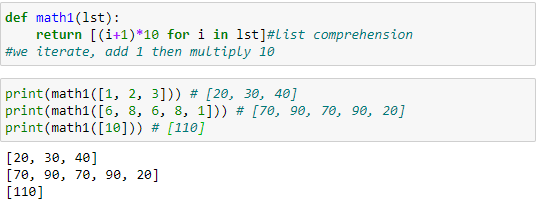#### Earn Coins

Coins can be redeemed for fabulous gifts.

Similar Homework Help Questions
• ### Python code that: Accepts a list of integers and an integer, and returns the index of...

Python code that: Accepts a list of integers and an integer, and returns the index of the SECOND  occurrence of the integer in the list. It returns None if the number does not occur two or more times in the list. Example 1: second_index([2,34,3,45,34,45,3,3], 3) returns 6 Example 2: second_index([2,34,3,45,34,45,3,3], 45) returns 5 Example 3: second_index([2,34,3,45,134,45,3,3], 134) returns None Example 4: second_index([2,34,3,45,134,45,3,3], 100) returns None

• ### In Python 5. Write a function named listComprehensionDivisors that has 3 integer inputs, N, n1, and...

In Python 5. Write a function named listComprehensionDivisors that has 3 integer inputs, N, n1, and n2. Using a single list comprehension to make a list of all of the numbers in the range 1..N that are either divisible by n1 or n2, where n1 and n2 can be any positive integers. After creating the list, your code should print out the following 2 lines: “We are printing all numbers from 1 to that are divisible by or ” “These...

• ### def second_index(a_list, number): Accepts a list of integers and an integer, and returns the index of...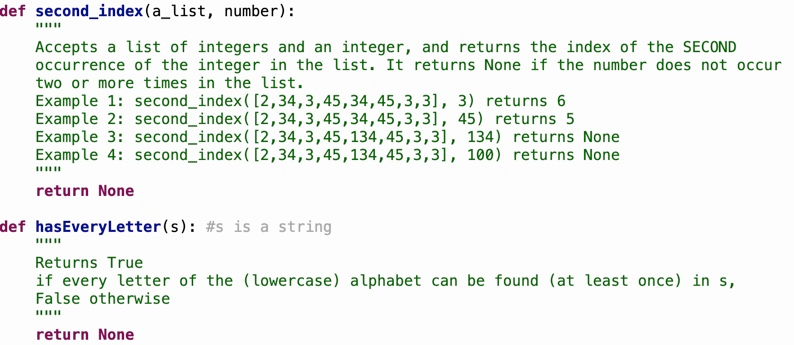def second_index(a_list, number): Accepts a list of integers and an integer, and returns the index of the SECOND occurrence of the integer in the list. It returns None if the number does not occur two or more times in the list. Example 1: second_index ([2,34,3,45,34,45,3,3), 3) returns 6 Example 2: second_index( [2,34,3,45,34,45,3,3), 45) returns 5 Example 3: second_index ( [2,34,3,45,134,45,3,3), 134) returns None Example 4: second_index( [2,34,3,45, 134,45,3,3), 100) returns None return None def hasEveryLetter(s): #s is a string Returns...

• ### in python 3 Create a list or tuple with 5 different integers. Prompt the user for...

in python 3 Create a list or tuple with 5 different integers. Prompt the user for an integer. If the number is in the list (or tuple) print a message stating the number was found. Otherwise, print a message stating the number was not found. Assume the list includes the numbers 0, 2, 4, 6, 8, and 10.

• ### Python: problem 1 Given an array of integers, return the sum of two indices from this...

Python: problem 1 Given an array of integers, return the sum of two indices from this array based on input parameters, x and y. Example: Given nums = [2, 7, 11, 15], x = 3, y = 1 Because nums[x] + nums[y] = 15 + 7 = 22, return 22. You may use this as a template for your code class Solution: def two_sum(self, nums: List[int], x: int, y: int) -> int:

• ### 1. use python List to Dictionary Write a function that has three parameters: a list of...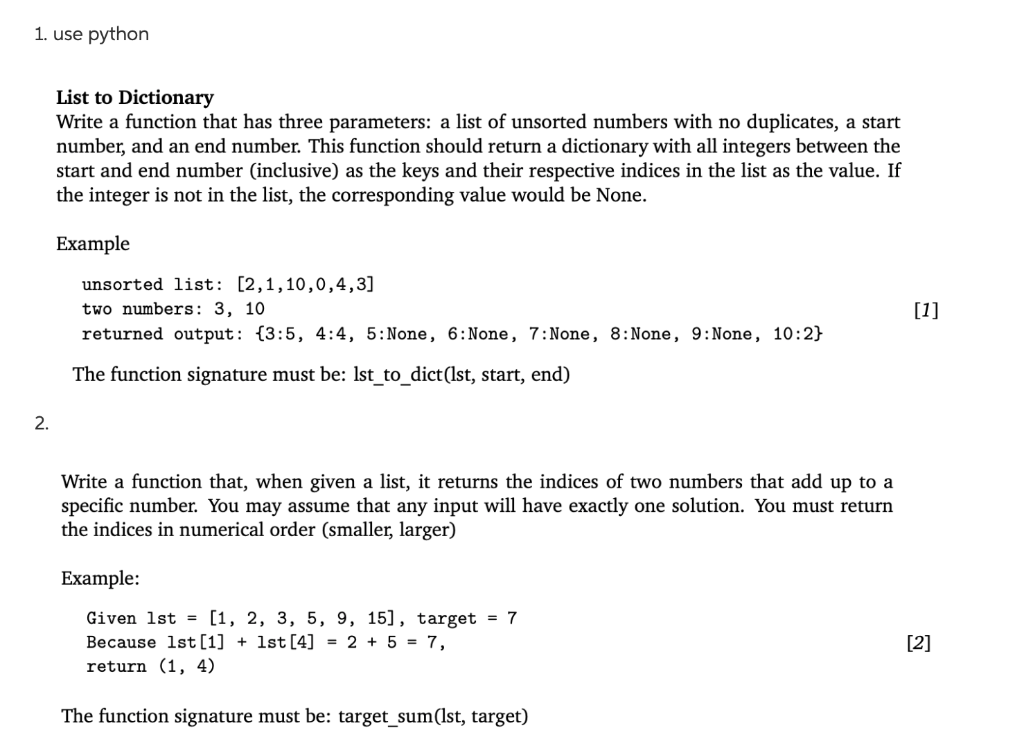1. use python List to Dictionary Write a function that has three parameters: a list of unsorted numbers with no duplicates, a start number, and an end number. This function should return a dictionary with all integers between the start and end number (inclusive) as the keys and their respective indices in the list as the value. If the integer is not in the list, the corresponding value would be None. Example unsorted list: [2,1,10,0,4,3] two numbers: 3, 10 returned...

• ### in Python please 6.8 LAB: Adjust values in a list by normalizing When analyzing data sets,...

in Python please 6.8 LAB: Adjust values in a list by normalizing When analyzing data sets, such as data for human heights or for human weights, a common step is to adjust the data. This can be done by normalizing to values between 0 and 1, or throwing away outliers. Write a program that first gets a list of integers from input. The input begins with an integer indicating the number of integers that follow. Then, adjust each integer in...

• ### Write a method called reverseFirstK that accepts an integer k and a queue of integers as...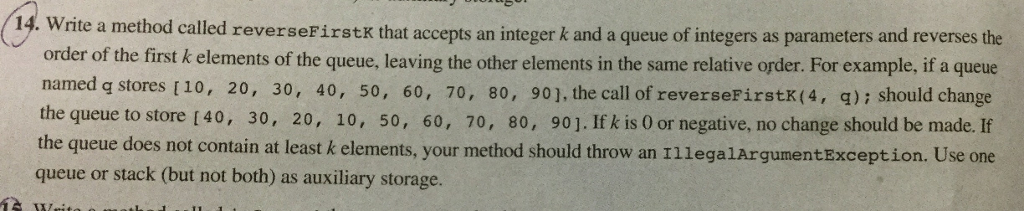Write a method called reverseFirstK that accepts an integer k and a queue of integers as parameters and reverses the order of the first k elements of the queue, leaving the other elements in the same relative order. For example, if a queue named q stores [10, 20 30, 40, 50, 60, 70, 80, 90], the call of reverseFirstK (4, q):should change the queue to store [40, 30 20, 10, 50, 60, 70, 80, 90]. If k is 0 or...

• ### Python Question: Define the function high_score that consumes a list of integers (representing scores in a...

Python Question: Define the function high_score that consumes a list of integers (representing scores in a game) and produces an integer representing the highest score in the list. Ignore scores less than 100, and stop processing values if you encounter -999. If the list is empty, return the value None instead. It is up to you to decompose this function (or not) however you want. Here is my code so far: from cisc108 import assert_equal def high_score(scores: [int])->int: max_num =...

• ### o Write a function that outputs even numbers less than the input number. • Function name...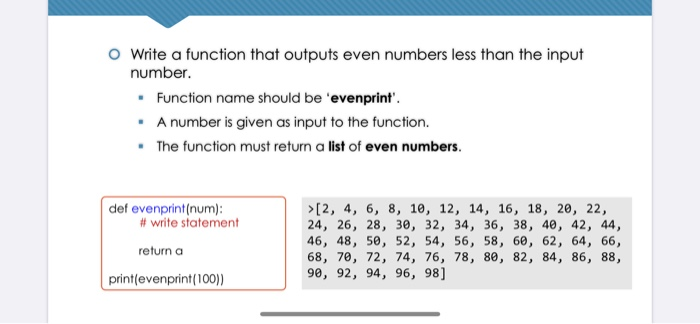o Write a function that outputs even numbers less than the input number. • Function name should be 'evenprint'. • A number is given as input to the function. . The function must return a list of even numbers def evenprint(num): #write statement >[2, 4, 6, 8, 10, 12, 14, 16, 18, 20, 22, 24, 26, 28, 30, 32, 34, 36, 38, 40, 42, 44, 46, 48, 50, 52, 54, 56, 58, 60, 62, 64, 66, 68, 70, 72, 74,...